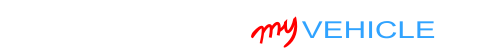## Interpreting The Compression Test Results

On high mileage engines, it's normal to see the compression value of each cylinder vary a bit. Depending on how much of a variation there is, you won't notice a difference in engine performance.

But if the compression values vary too much, then your mini-van's 2.5L 4 cylinder engine will suffer a rough idle or a misfire problem.

The key to finding out is to do some math and see if the lowest compression value you got differs by more than 15% of the highest value.

You can do this by using my online low compression calculator here: Online Low Engine Compression Calculator (at: easyautodiagnostics.com).

Or you can calculate this 15% difference, with pen and paper, like this:

1. STEP 1: Multiply the highest compression value by 0.15 (this is the decimal value of 15%).
2. STEP 2: Round the result to the nearest one (for example: 25.6 would become 26).
3. STEP 3: Subtract the result (the number that was rounded) from the highest compression value.
4. ANSWER: The result of this subtraction is the lowest possible compression value any cylinder can have.

To explain how to figure out this 15% thing manually, let's say that I got the following compression test results from a 2.5L Dodge Caravan:

Cylinder Pressure
#1 175
#2 160
#3 170
#4 110

My next step is to do the following calculation:

1. STEP 1:  175 x 0.15 = 26.25.
2. STEP 2:  26.25 = 26 (rounded to nearest one).
3. STEP 3:  175 - 26 = 149.
4. ANSWER:  149 PSI. Any cylinder with this compression (or lower) value will misfire.

Since cylinder #4 is only producing 110 PSI, I can now conclude that it's 'dead'.

To find out if the lowest compression value you got from your engine compression test is within a good range, you'll need to do the same calculation. Of course, you'll need to use the highest compression value you got and not the one in the example.

Once you've found the 'dead' cylinder, the next step is to find out what's causing the low compression value. For this step, go to: TEST 2: Wet Compression Test.

## TEST 2: Wet Engine Compression TestA 'wet' compression test will help you find out what's causing the low or 0 PSI compression value of a cylinder with a compression issue.

To do a 'wet' compression test, 2 tablespoons of engine oil are added to the cylinder with low or 0 PSI compression. Then the cylinder's compression is tested again.

If the compression value increases, from the value you got in TEST 1, then you can conclude that the low compression problem of that cylinder is due to bad piston rings.

If the compression value does not increase, then you can conclude that that cylinder's compression problem is due to bad or damaged cylinder head valves.

These are the test steps:

1. 1

Add 1 or 2 tablespoon of engine oil to the cylinder you need to retest.

Use a small and long funnel so that the oil will reach the inside of the cylinder.

2. 2

Install the compression gauge on the cylinder you just added oil to.

3. 3

Have your helper crank the engine till the needle stops climbing on the compression gauge.

4. 4

You'll see one of two results:

1.) The needle will climb higher than the previous compression number you recorded for this specific cylinder, or...

2.) The needle will not move at all or stay at the same number you recorded earlier.

5. 5

Repeat steps 1 thru' 4 on any other cylinder you need to check.

CASE 1: The compression value increased. This test result tells you that the low or near 0 PSI compression value is due to worn out piston rings of that specific cylinder.

CASE 2: The compression value DID NOT increase. This test result confirms that the cylinder head valves of that cylinder are worn-out or damaged.

## More 2.5L Dodge Caravan And Plymouth Voyager Tutorials

You can find all of the 2.5L Dodge Caravan and 2.5L Plymouth Voyager tutorials in this index:

Here's a small sample of the articles/tutorials you'll find in the index:If this info saved the day, buy me a beer!#### Latest

﻿

Dodge Vehicles:

• Caravan 2.5L
• 1991, 1992, 1993, 1994, 1995

Plymouth Vehicles:

• Voyager 2.5L
• 1991, 1992, 1993, 1994, 1995